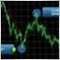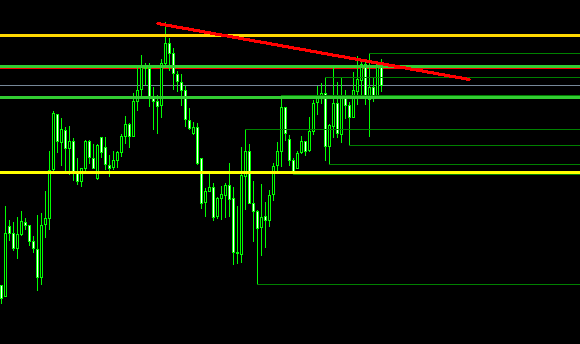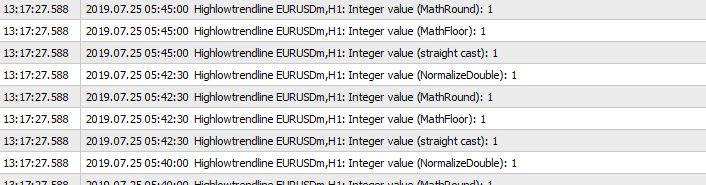# How can get double value of previous day high and 4hr high to draw trendline in 1hour chart?184

Hello every coders

Good day to you all.

I need some idea help.

As we know trendline object create use only int value and bar count.

I get problem when I try to draw a trendline from previous day high double value to 4hour high double value in 1hour timeframe.Please see picture. I want to draw like this.

Yellow line is previous day high and green line is 4hour high  and red trendline is to connect this swing point to draw trendline to confirm that is downtrend.

Here is my codes

```   double yesterdayHigh = iHigh(NULL,PERIOD_D1,1);
double yesterdayLow  = iLow(NULL, PERIOD_D1,1);

double Prev4HHigh = iHigh(NULL,PERIOD_H4,1);
double Prev4HLow  = iLow(NULL, PERIOD_H4,1);

```

No trendline on chart because double value change to int get only bar count (example 0 or 1).

This cannot be use in 1hour time frame

I know how to change double to int value as follow.

```   int integer_value_straight_cast = yesterdayHigh; // Rounds towards zero (same as MathFloor() for positive numbers, different for negative numbers)
int integer_value_MathFloor = MathFloor(yesterdayHigh);   // Rounds down
int integer_value_MathRound = MathRound(yesterdayHigh);   // Rounds to the nearest integer
int integer_value_NormalizeDouble = NormalizeDouble(yesterdayHigh, 0);   // Effectively the same as MathRound()

Print("Integer value (straight cast): " + integer_value_straight_cast);  // Displays -9
Print("Integer value (MathFloor): " + integer_value_MathFloor);  // Displays -10
Print("Integer value (MathRound): " + integer_value_MathRound);  // Displays -10
Print("Integer value (NormalizeDouble): " + integer_value_NormalizeDouble);  // Disp```

But this output 1 0r 0 not useful.How can draw trendline from previous day high double value to 4hr high double value in object create function.2. ```ObjectCreate("buyline",OBJ_TREND,0,Time[(int)yesterdayHigh],High[(int)yesterdayHigh],Time[(int)Prev4HHigh],High[(int)Prev4HHigh]);